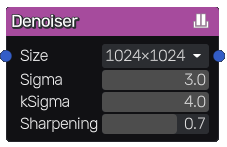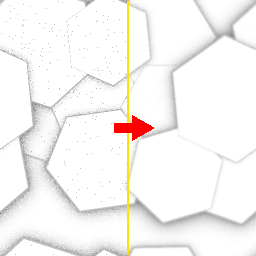# Denoiser node¶

The Denoiser node performs fast spatial deNoise filter, with circular gaussian kernel.## Inputs¶

The Denoiser node accepts one input:

• The Source inputs is the image to be denoised.

## Outputs¶

The Denoiser node outputs the denoised image.

### Parameters¶

The Denoiser node has the following parameters:

• the Size determines the resolution of the denoiser

• the Standard Deviation Sigma radius

• the kSigma is the K factor sigma coefficient:
• kSigma = 1*sigma cover 68% of data

• kSigma = 2*sigma cover 95% of data - but there are over 3 times more points to compute

• kSigma = 3*sigma cover 99.7% of data - but needs more than double the calculations of 2*sigma

• the edge Sharpening threshold

### Example images¶